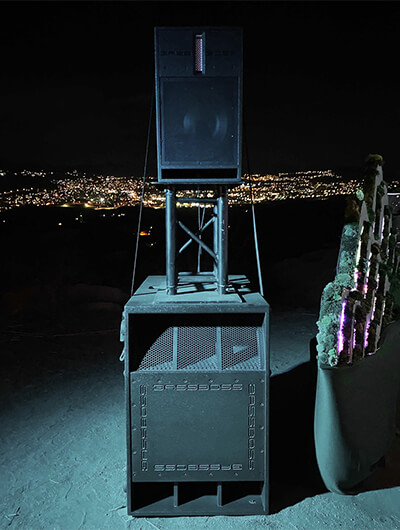# Sensitivity = Efficiency in Speakers?

April 28, 2019Sensitivity is the output sound pressure level that is measured at 1m when 1 Watt of power is being consumed. The original standard specified 1W, which required converting to volts according to the loudspeaker’s nominal impedance. At 8-ohms, 1 Watt would be with 2.83V applied. At 4 ohms, 1 Watt would require only 2V. The current standard allows using 2.83V into the loudspeaker, regardless of its impedance. This causes some confusion about what is being specified as Sensitivity.

Efficiency is the ratio of acoustical output power to electrical input power, measured in watts, expressed as a percentage. The measurement is taken at 1m from the loudspeaker.

If you convert sensitivity to efficiency, assuming an 8-ohm speaker and 2.83V applied, you will find that a loudspeaker with a sensitivity of 92dB is 1% efficient. That’s right, 1%. For each 3dB increase in sensitivity, the efficiency doubles. So 95dB sensitivity is 2% efficiency and so on.

To find the efficiency of a 4-ohm speaker specified using 2.83V input, you simply subtract 3dB from the specified sensitivity and do the conversion. For a 2-ohm speaker, you subtract 6dB. For a 16-ohm speaker you would add 3dB.

Expressed in words, some of the resulting truths sound pretty bad: For a speaker with a sensitivity of 82dB, one-tenth of one percent of the electrical power applied to the speaker is realized as acoustical power at 1m from the speaker.

Just to put it all in perspective, one Acoustical Watt is realized at a sound pressure level of 112dB.